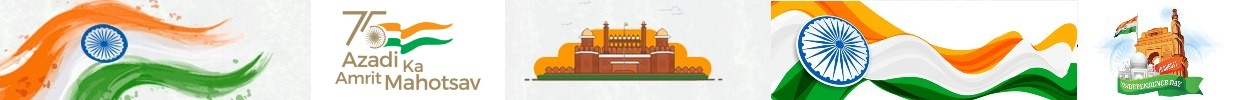## RELATED POSTS

Father has made a couple of dice. Unni and friends play with it.
Look at what unni got?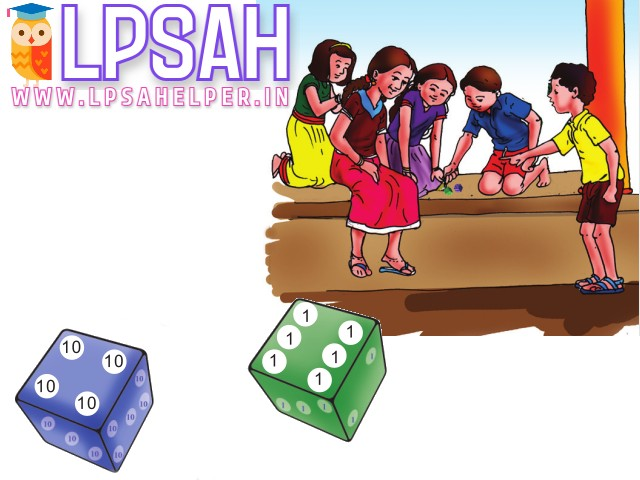How many points for him?
4 tens on the blue dice = 10 + 10 + 10 + 10 = 40
6 ones on the green dice = 1 + 1 + 1 + 1 + 1 + 1 = 6
40 and 6 make 46
Unni got 46 points.
Find others points and write them.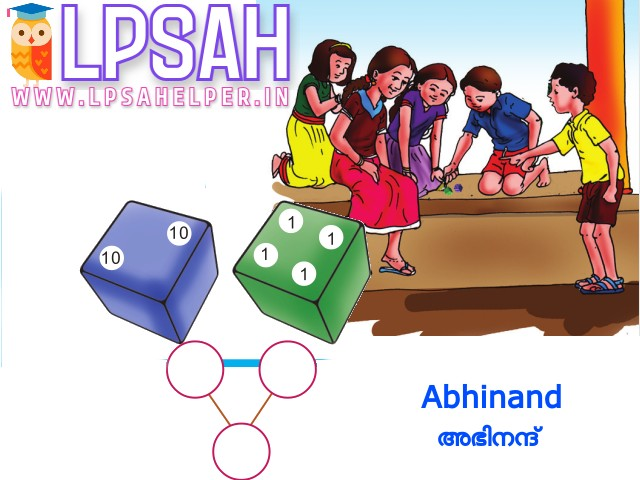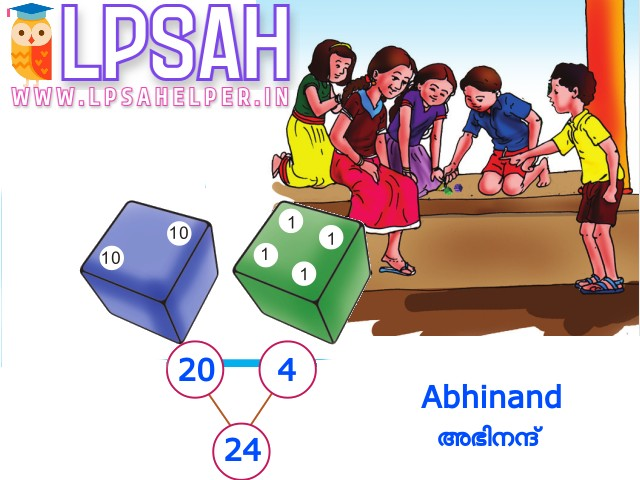How many points for him?
2 tens on the blue dice = 10 + 10 = 20
4 ones on the green dice = 1 + 1 + 1 + 1 = 4
20 and 4 make 24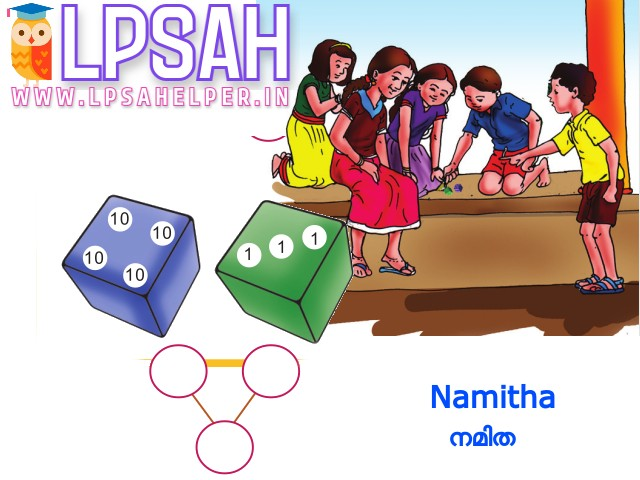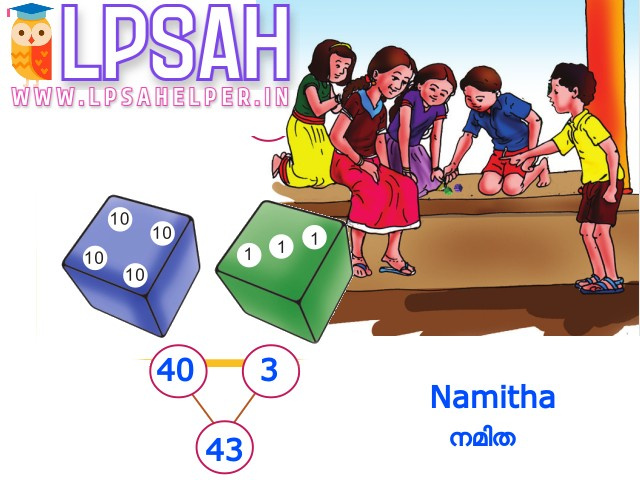How many points for her?
4 tens on the blue dice = 10 + 10 + 10 + 10 = 40
3 ones on the green dice = 1 + 1 + 1 = 3
40 and 3 make 43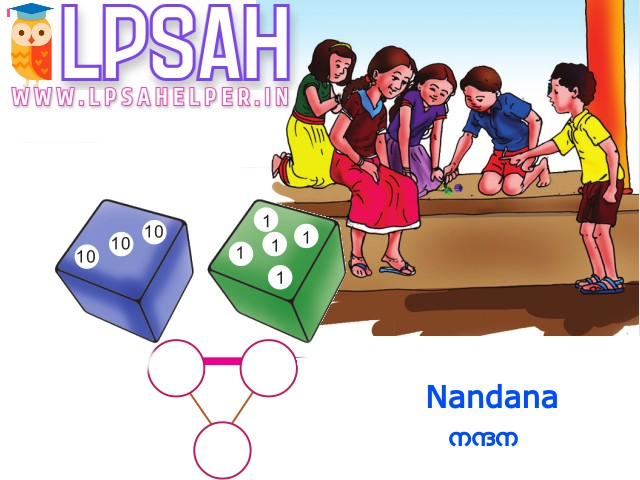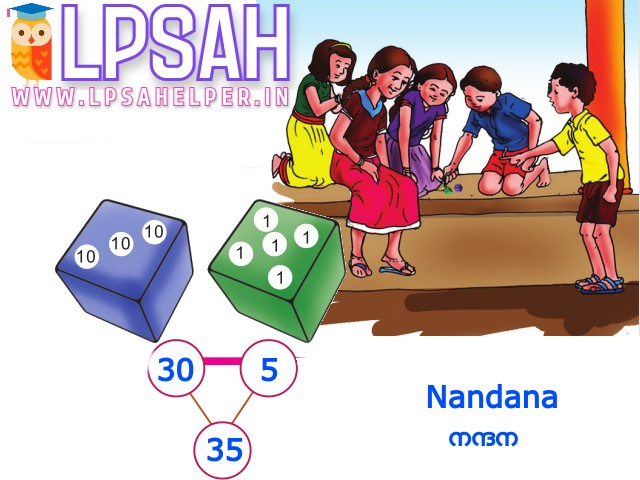How many points for her?
3 tens on the blue dice = 10 + 10 + 10 = 30
5 ones on the green dice = 1 + 1 + 1 + 1 + 1 = 5
30 and 5 make 35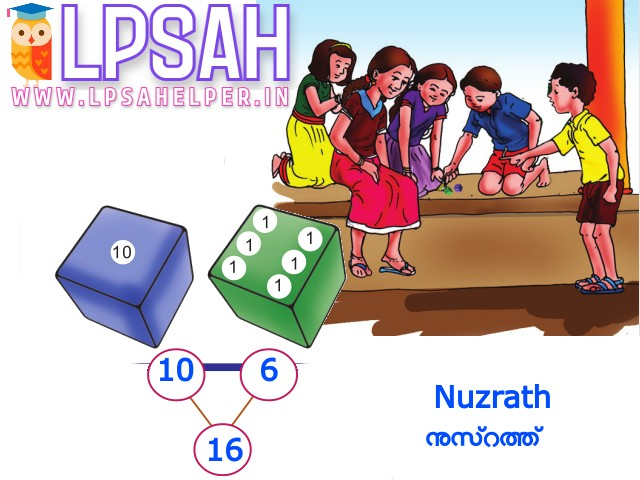How many points for her?
1 tens on the blue dice = 10 = 10
6 ones on the green dice = 1 + 1 + 1 + 1 + 1 + 1 = 6
10 and 6 make 16
First place for the person who got most points.
Second place for the next and so on.
Write them in order, from most to least
And the names too!
 POSITION ONE TWO THREE FOUR FIVE NAME Unni Namitha Nandana Abhinand Nuzrath POINT 46 43 35 24 16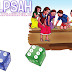Share it: